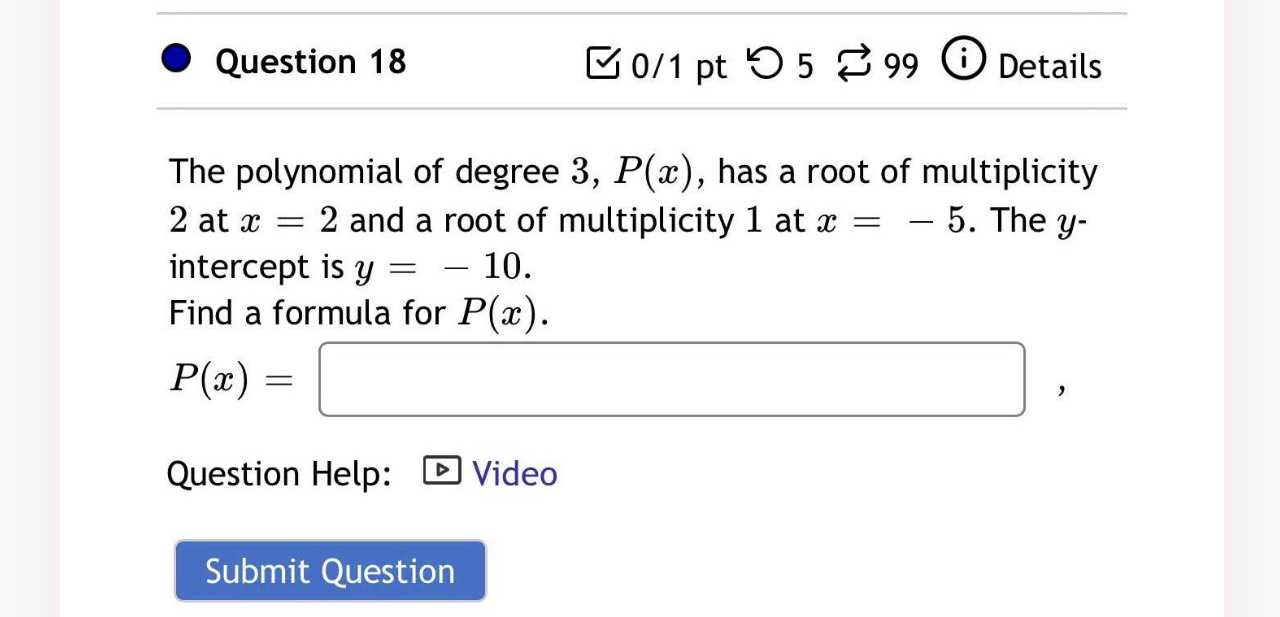### Still have math questions?

Algebra
QuestionQuestion $$18$$ The polynomial of degree $$3 , P ( x )$$ , has a root of multiplicity

$$2$$ at $$x = 2$$ and a root of multiplicity $$1$$ at $$x = - 5$$ . The $$y$$ - intercept is $$y = - 10$$ . Find a formula for $$P ( x )$$ .

$$P ( x ) = \square$$ Question Help: $$\square$$ Video Submit Question

p(x) = ($$\frac{- 1}{2}$$)(x-2)$$^{2}$$(x+5)